Newton’s laws of motionPage 4

WATCH ALL SLIDES

Slide 27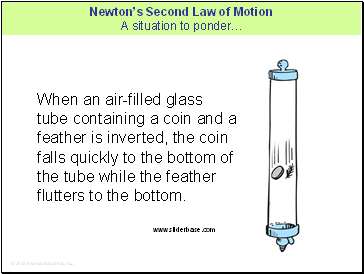Newton’s Second Law of Motion A situation to ponder…

When an air-filled glass tube containing a coin and a feather is inverted, the coin falls quickly to the bottom of the tube while the feather flutters to the bottom.

Slide 28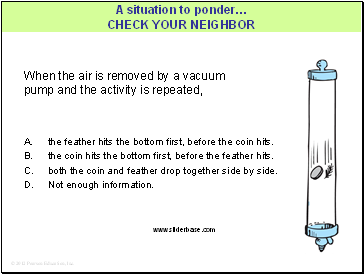When the air is removed by a vacuum pump and the activity is repeated,

A. the feather hits the bottom first, before the coin hits.

the coin hits the bottom first, before the feather hits.

both the coin and feather drop together side by side.

Not enough information.

A situation to ponder…

Slide 29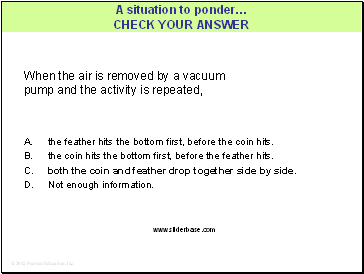When the air is removed by a vacuum pump and the activity is repeated,

A situation to ponder…

A. the feather hits the bottom first, before the coin hits.

the coin hits the bottom first, before the feather hits.

both the coin and feather drop together side by side.

Not enough information.

Slide 30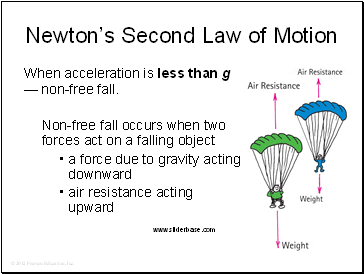Newton’s Second Law of Motion

When acceleration is less than g — non-free fall.

Non-free fall occurs when two forces act on a falling object

a force due to gravity acting downward

air resistance acting upward

Slide 31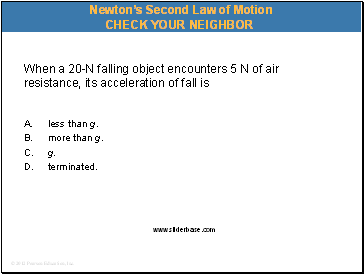When a 20-N falling object encounters 5 N of air resistance, its acceleration of fall is

A. less than g.

more than g.

C. g.

terminated.

Newton’s Second Law of Motion

Slide 32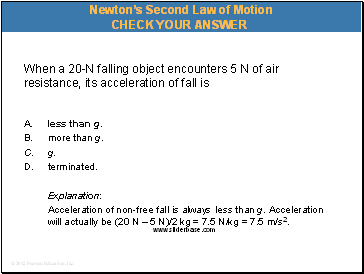When a 20-N falling object encounters 5 N of air resistance, its acceleration of fall is

A. less than g.

more than g.

g.

terminated.

Explanation:

Acceleration of non-free fall is always less than g. Acceleration will actually be (20 N – 5 N)/2 kg = 7.5 N/kg = 7.5 m/s2.

Newton’s Second Law of Motion

Slide 33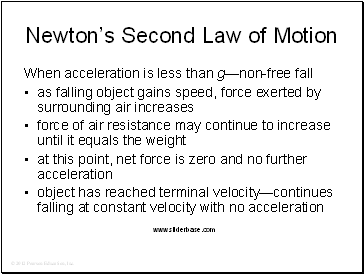Newton’s Second Law of Motion

When acceleration is less than g—non-free fall

as falling object gains speed, force exerted by surrounding air increases

force of air resistance may continue to increase until it equals the weight

at this point, net force is zero and no further acceleration

object has reached terminal velocity—continues falling at constant velocity with no acceleration

Slide 34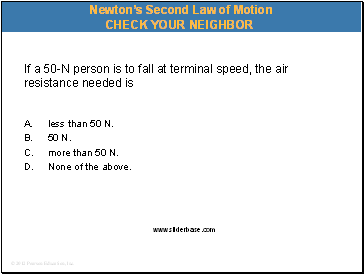If a 50-N person is to fall at terminal speed, the air resistance needed is

Go to page:
1  2  3  4  5  6  7  8  9